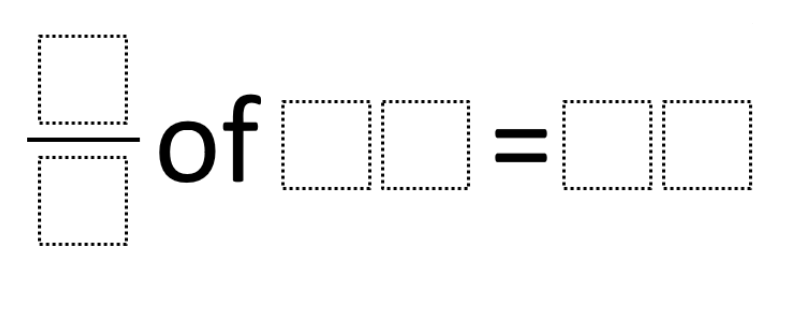# Fraction of an Amount

Directions: Using the digits from 1 to 9, at most once, make as many true statements as possible.### Hint

1/2 of 68 = 34
6/3 of 27 = 54

Source: Rochelle Telfer

## Solving One-Step Inequalities with Addition

Directions: Using the digits 0 to 9 at most one time each, place a digit …

1.i dont know what i comment about but at first i was super confused but then i saw 34 plus 34 equals 68 same with the numbers below so you just multiply the number you equal by 2

2.6/3 of 27=54

3.1/2 of 68=34

6/3 of 27 =54

•6/3 of 27 =54

4.4/2 x 18 = 36

5.1/4 of 8=2

6.1/2 of 68=34
1/4 of 8=2
Or
6/3 of 27 =54 4/2 x 18 = 36

7.1/2 of 16 is 8
1/8 of 56 is 7

8.1/3 of 6 is 2
1/2 Of 8 is 4

9.10.1/2 of 68=34
or
6/3 of 27=54

11.Elizabeth Bender

1/2 of 8 is 4
1/2 of 68 is 34
1/3 of 6 is 2
2/3 of 12 is 8

12.4/8=1/2
2/4=1/2
5/10=1/2
3/6=1/2

13.14.2/3 of 12 = 8
2/3 of 6 = 4
1/4 of 8= 2
1/2 of 6 = 3
1/2 of 8 = 4

15.Harrison McMurray

9/6 of 18 =27

16.2/3 of 6= 4

17.1/2 of 68=34
6/3 of 27=54

18.why are they giving us the answer?

19.2/4 of 28=14

20.Corben Gullinese

1\9 of 81=9

21.1/2 of 96= 48

22.1/2 of 43=21.5

23.½ of 6=3
4/2 of 7=14

24.1/2=88=44=1/2=22=1/2=11

25.26.1/2 of 30=15

27.1/9 of 91 = 9.91

28.1/4 of 100 is 25
1/3 of 9 is 3

29.1/2 of 68=34

30.3/4 of 250 =

31.8/10 of 250 = 200

•8/10 of 250 = 200

32.4/8 of 64 is = 32

33.34.Claire Powell (;

1/2 of 72= 36

35.6/3 of 27=54
1/2 of 72=36

36.Danitza Marenco

8/10 of 250=200

37.1/2 of 68 = 34
6/3 of 27 = 54

38.Careful about your answers. The equation we are answering has 2 blank digits after the OF and after the =, and 0 (zero) is NOT one of the allowed numbers we can use to complete the equation. Many of the solutions above contain 0’s or do not fill in all the blank boxes…..

39.1/2 of 72 is 36

40.2/1 of 34=68

41.1/2 of 61=30.5
1/2 of 76=38
1/2 of 86=43
1/2 of 96=48

42.There are 120 correct solutions for this problem. I have included them all here:

1/2*68=34
1/2*76=38
1/2*78=39
1/2*86=43
1/2*96=48
1/3*78=26
1/3*87=29
2/1*34=68
2/1*38=76
2/1*39=78
2/1*43=86
2/1*48=96
2/3*81=54
2/3*84=56
2/4*36=18
2/4*38=19
2/4*76=38
2/4*78=39
2/6*54=18
2/6*57=19
2/7*63=18
2/8*56=14
2/8*76=19
2/9*63=14
3/1*26=78
3/1*29=87
3/2*54=81
3/2*56=84
3/4*68=51
3/4*96=72
3/6*28=14
3/6*54=27
3/6*58=29
3/6*82=41
3/7*42=18
3/7*49=21
3/7*56=24
3/7*98=42
3/8*56=21
3/9*48=16
3/9*54=18
3/9*78=26
3/9*81=27
4/2*18=36
4/2*19=38
4/2*38=76
4/2*39=78
4/3*51=68
4/3*72=96
4/6*27=18
4/6*57=38
4/6*78=52
4/7*28=16
4/7*56=32
4/7*91=52
4/7*98=56
4/8*26=13
4/8*32=16
4/8*62=31
4/8*72=36
4/9*63=28
4/9*81=36
6/2*18=54
6/2*19=57
6/3*14=28
6/3*27=54
6/3*29=58
6/3*41=82
6/4*18=27
6/4*38=57
6/4*52=78
6/8*52=39
6/8*72=54
6/9*27=18
6/9*48=32
6/9*51=34
6/9*57=38
6/9*72=48
6/9*78=52
6/9*81=54
7/2*18=63
7/3*18=42
7/3*21=49
7/3*24=56
7/3*42=98
7/4*16=28
7/4*32=56
7/4*52=91
7/4*56=98
7/8*56=49
7/9*36=28
7/9*81=63
8/2*14=56
8/2*19=76
8/3*21=56
8/4*13=26
8/4*16=32
8/4*31=62
8/4*36=72
8/6*39=52
8/6*54=72
8/7*49=56
8/9*72=64
9/2*14=63
9/3*16=48
9/3*18=54
9/3*26=78
9/3*27=81
9/4*28=63
9/4*36=81
9/6*18=27
9/6*32=48
9/6*34=51
9/6*38=57
9/6*48=72
9/6*52=78
9/6*54=81
9/7*28=36
9/7*63=81
9/8*64=72

43.1/2 of 68 = 34
6/3 of 27 = 54

44.1/2 of 68 = 34

45.1/2 of 42 = 21
3/7 of 21 = 9

46.6/3 of 27=54

47.2/4 of 36 =18

48.1-2 of 68=34
6-3 of 27=54

49.7/9 of 81=63
Gabrielle St Oran’s College
My fellow genius classmate